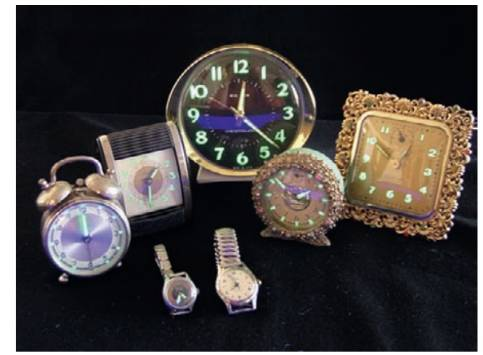Chapter 19.3, Problem 19.3SC### Introductory Chemistry: A Foundati...

9th Edition
Steven S. Zumdahl + 1 other
ISBN: 9781337399425

#### Solutions

Chapter
Section### Introductory Chemistry: A Foundati...

9th Edition
Steven S. Zumdahl + 1 other
ISBN: 9781337399425
Textbook Problem
19 views

# Exercise19.3Watches with numerals that glow in the dark” formerly were made by including radioactive radium in the paint used to letter the watch faces. Assume that to make the numeral 3 on a given watch, a sample of paint containing 8 .0 × 1 0 − 7 mole of R 88 228 a was used. This watch was then put in a drawer and forgotten. Many years later someone finds the watch and wishes to know when it was made. Analyzing the paint, this person finds 1.0 × 1 0 − 7 moles of R 88 228 a in the numeral 3. Flow much time elapsed between the making of the watch and the finding of the watch?Watches and clocks with radium dials.

Interpretation Introduction

Interpretation:

Interpret the elapsing time for the finding of watch if initial number of mole of Ra present in watch was × 10-7mol while the present number of mole is 10-7 mole.

Concept Introduction:

The time elapsed can be identified through the below formula:

(N /N0) = (1 /2)t /t half

N is number of mole left after time t

N0 is initial number of mole

Th a l f is half life.

Explanation

For determining time elapsed,

(N /N0) = (1 /2)t /t halfN = 1 × 10-7molN0= 8 × 10-7molT half = 1600&#

### Still sussing out bartleby?

Check out a sample textbook solution.

See a sample solution

#### The Solution to Your Study Problems

Bartleby provides explanations to thousands of textbook problems written by our experts, many with advanced degrees!

Get Started

#### Find more solutions based on key concepts